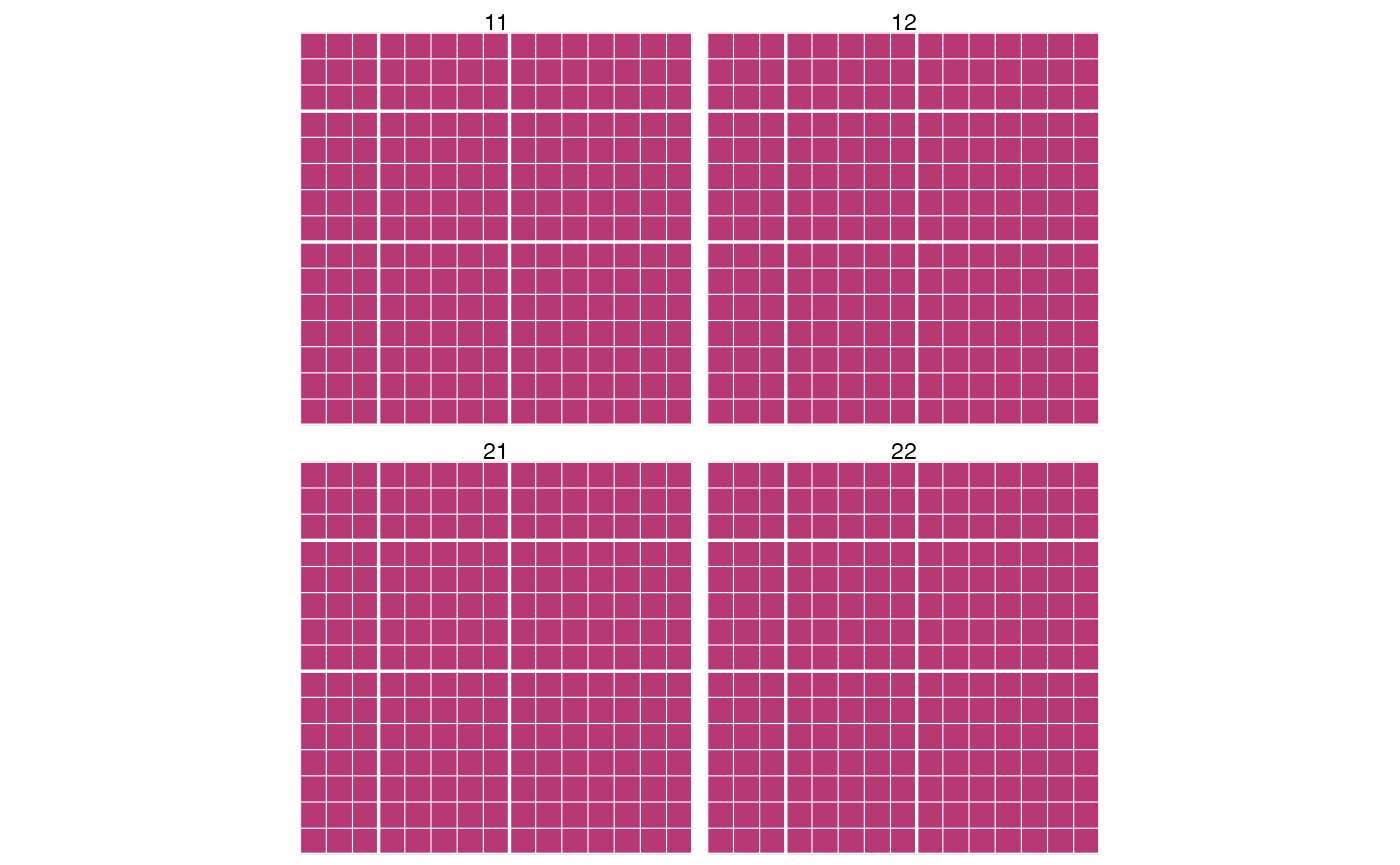## Single particle T-matrix

The convention is to use

• m: multipole order, -n:n
• n: multipole degree 1:nmax
• q: multipole type, 1 or 2

so for a T-matrix with Nmax=3, we have

## n: 1..3
## m: -3..3
## q: 1..2
## 900 elementsfor each block we introduce a combined p-index such that,

$p(n,m) = n(n+1)+m$ which here varies as p: 1..15, as each block is of dimension pmax = nmax(nmax+1)+nmax = 3(3+1)+3 = 15.

The whole T-matrix is indexed with a combined l-index such that,

$l(q,p) = (q-1)p_{max} + p$ which here gives us, l: 1..30. The total T-matrix dimension (along rows/columns) is thus $$l_{max}=2\times(nmax(nmax+1)+nmax)=30$$.

In summary, for a given $$N_{max}$$ the indices are given by:

• $$n=1:N_{max}$$
• $$m=-n:n$$ for each n
• $$q=1:2$$ for each pair of (n,m)
• $$p(n,m) = n(n+1)+m$$
• $$l(q,p) = (q-1)p_{max} + p$$

Given $$l$$ and $$N_{max}$$ we can invert these indices as follows,

• $$p = l - (q-1)l_{max}/2$$,
giving here, 1, 2, 3, 4, 5, 6, 7, 8, 9, 10, 11, 12, 13, 14, 15, 1, 2, 3, 4, 5, 6, 7, 8, 9, 10, 11, 12, 13, 14, 15
• $$n = \lfloor\sqrt{p}\rfloor$$,
giving here 1, 1, 1, 2, 2, 2, 2, 2, 3, 3, 3, 3, 3, 3, 3, 1, 1, 1, 2, 2, 2, 2, 2, 3, 3, 3, 3, 3, 3, 3
• $$m = p - n(n+1)$$,
giving here -1, 0, 1, -2, -1, 0, 1, 2, -3, -2, -1, 0, 1, 2, 3, -1, 0, 1, -2, -1, 0, 1, 2, -3, -2, -1, 0, 1, 2, 3
1 2 3 4 5 6 7 8 9 10 11 12 13 14 15 16 17 18 19 20 21 22 23 24 25 26 27 28 29 30
p 1 2 3 4 5 6 7 8 9 10 11 12 13 14 15 1 2 3 4 5 6 7 8 9 10 11 12 13 14 15
n 1 1 1 2 2 2 2 2 3 3 3 3 3 3 3 1 1 1 2 2 2 2 2 3 3 3 3 3 3 3
m -1 0 1 -2 -1 0 1 2 -3 -2 -1 0 1 2 3 -1 0 1 -2 -1 0 1 2 -3 -2 -1 0 1 2 3

## Multi-particle matrices

When combining multiple particles we introduce an additional index $$j=1:j_{max}$$ tracking the particle number, and a combined index $$i$$, $i = (j-1) l_{max} + l.$

Knowing the number of particles, $$j_{max}$$, and the maximum order $$n_{max}$$, we can unpack the full set of indices with the following formulas,

• $$j = (i-1) / (i_{max}/j_{max}) + 1$$ (integer division)
• $$l = i - (j-1)\times i_{max}/j_{max}$$ (integer division)
• $$q = 2(l-1)/l_{max} + 1$$ (integer division)
• $$p = l - (q-1)l_{max}/2$$
• $$n = \lfloor\sqrt{p}\rfloor$$
• $$m = p - n(n+1)$$